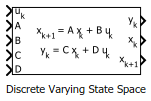# Discrete Varying State Space

Discrete-time state-space model with varying matrix values

•Libraries:
Control System Toolbox / Linear Parameter Varying

## Description

Use this block to implement a discrete-time state-space model with varying matrices. Feed the instantaneous values of the state matrix A, input matrix B, output matrix C, and feedforward matrix D to the corresponding input ports. The system response is given by:

`$\begin{array}{c}{x}_{k+1}=A{x}_{k}+B{u}_{k}\\ {y}_{k}=C{x}_{k}+D{u}_{k},\end{array}$`

where uk is the system input, yk is the system output, xk is the current system state, and xk+1 is the system state at the next time step.

Use this block and the other blocks in the Linear Parameter Varying library to implement common control elements with variable parameters or coefficients. For more information, see Model Gain-Scheduled Control Systems in Simulink.

Caution

Avoid making the C and D matrices depend on the system output yk. If you have such dependence, the resulting state-space equation yk = C(yk)xk + D(yk)uk creates an algebraic loop, because computing the output value yk requires knowing the output value. This algebraic loop is prone to instability and divergence. Instead, try expressing C and D in terms of the time t, the block input uk, and the state outputs xk.

For similar reasons, avoid making A and B depend on the xk+1 output. Note that it is safe to for A and B to depend on yk when yk is a fixed combination of states and inputs, (in other words, when yk = Cxk + Duk where C and D are constant matrices).

## Ports

### Input

expand all

System input signal.

State matrix of dimensions Nx-by-Nx, where Nx is the number of system states.

Input matrix of dimensions Nx-by-Nu, where Nu is the number of system inputs.

Output matrix Ny-by-Nx, where Ny is the number of system outputs.

Feedforward matrix of dimensions Ny-by-Nu.

For a zero-feedthrough state-space model, clear the Enable feedthrough checkbox. Clearing this checkbox disables the D input port. (since R2023a)

### Output

expand all

System output signal.

Current state values.

#### Dependencies

To enable this port, select the Output states parameter.

State values at next time step.

#### Dependencies

To enable this port, select the Output state updates parameter.

## Parameters

expand all

Initial state values, specified as a scalar or a vector whose length is the number of system states.

Block sample time, specified as either –1 (inherited sample time) or a positive scalar value.

Select to enable the state values output port, x.

Select to enable the state updates output port, xk+1.

Since R2023a

Enable the D input port for a state-space model with direct feedthrough. For a zero-feedthrough model, clear this checkbox. Disabling the port for zero-feedthrough models is numerically more reliable than feeding a zero-constant into the port.

## Version History

Introduced in R2017b

expand all